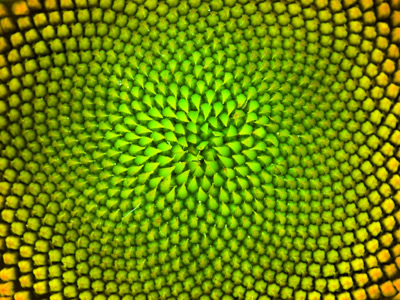The pattern formed in this flower relates to a number sequence called the Fibonacci Sequence.

# Sequences

This Math quiz is called 'Sequences' and it has been written by teachers to help you if you are studying the subject at middle school. Playing educational quizzes is a fabulous way to learn if you are in the 6th, 7th or 8th grade - aged 11 to 14.

It costs only \$12.50 per month to play this quiz and over 3,500 others that help you with your school work. You can subscribe on the page at Join Us

A sequence, or number pattern, is a list of numbers that follow a rule. The most basic type of sequence comes from adding (or subtracting) the same number each time, but more complex patterns can be formed by multiplying, squaring, increasing the number that is added, and so on. See how you can spot the pattern in this middle school Math quiz.

Trying to spot the rule in a number pattern requires a bit of detective work. First, work out the difference between consecutive terms. If the difference is always the same, then you know the rule has something to do with this times table. For example, if the difference is always 4, then the rule is linked to the 4 times table, which we write as 4n.

The next stage is to compare this times table with your number pattern. Ask yourself, what do you do to the times table to get the number pattern?

If the answer is ‘add 3’, then add this to your rule. This will give a rule that looks something like 4n + 3.

Writing the rule for more complex patterns can be quite hard, but it is often easy to spot what is going on. A famous pattern is the Fibonacci sequence, where each successive term is created by adding the two previous. This sequence appears in many forms in nature, such as the pattern formed in sunflower seeds or the scales on a pineapple.

Question 1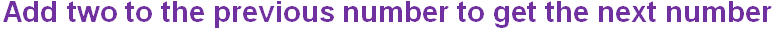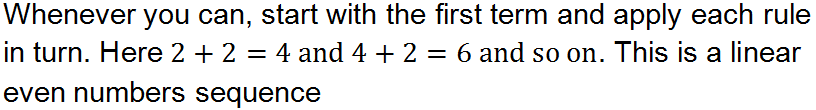Question 2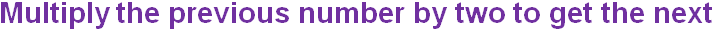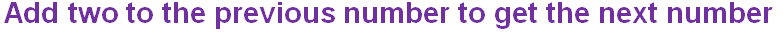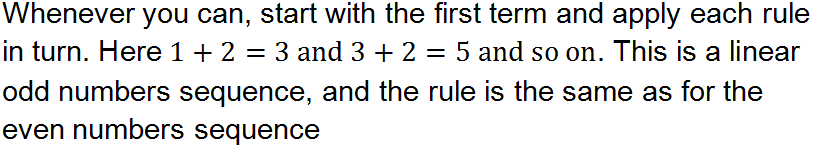Question 3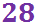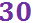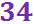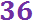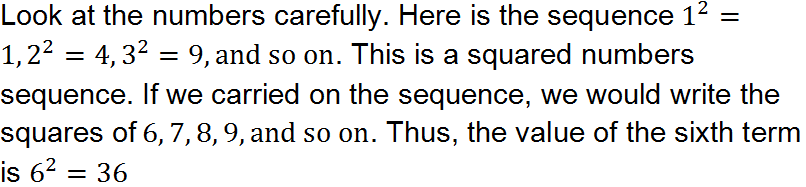Question 4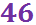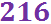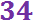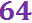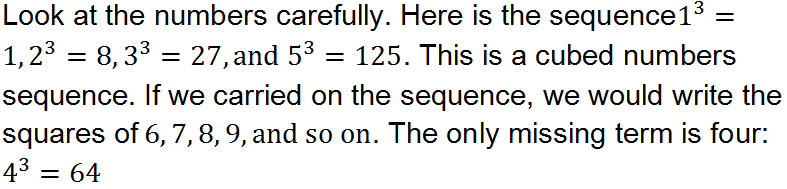Question 5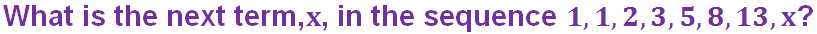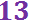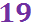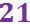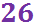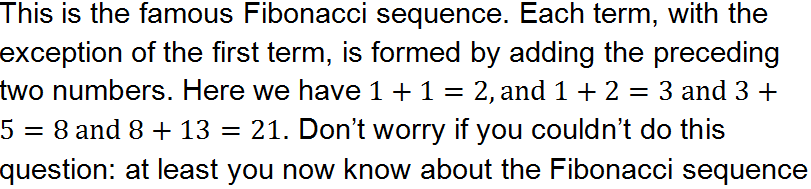Question 6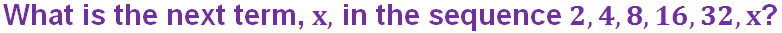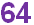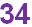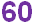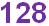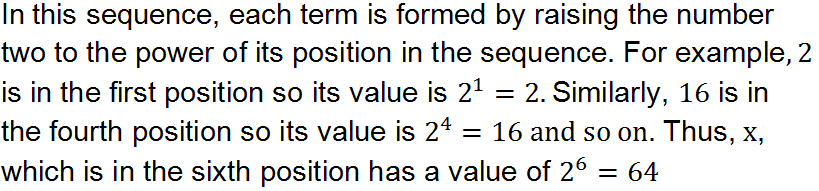Question 7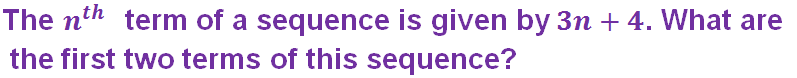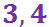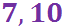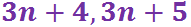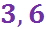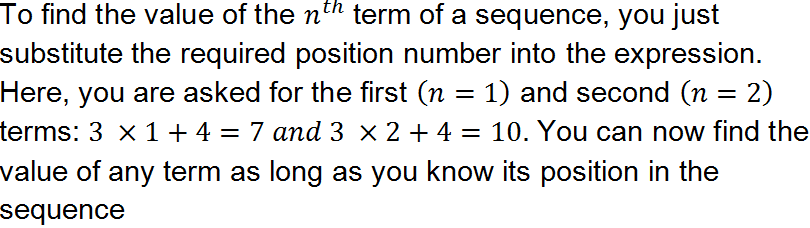Question 8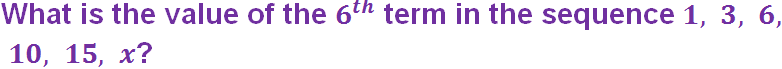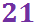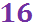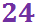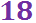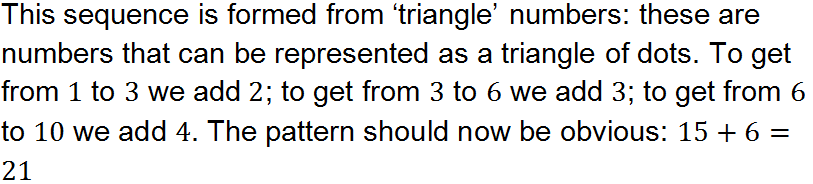Question 9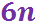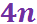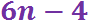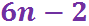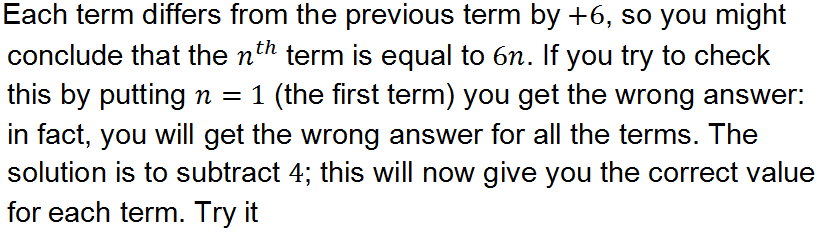Question 10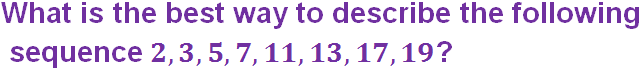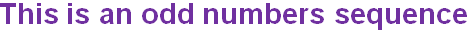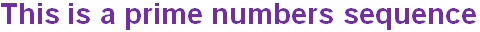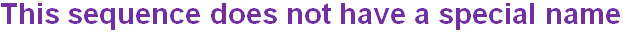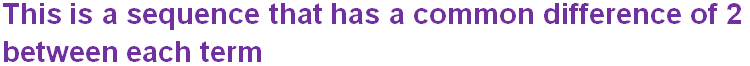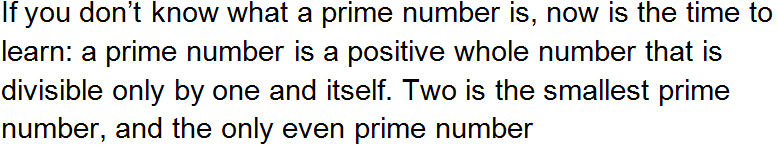Author:  Frank Evans